Do you like the article?
Share it with others -

Use new possibilities of MetaTrader 5

Transferring Indicators from MQL4 to MQL5

28 July 2010, 10:44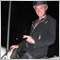3
19 086

Introduction

In today's article I'm going to represent you an algorithm of transferring simple price calculations from MQL4 to MQL5. With a glance to the difference between MQL5 and MQL4 I added the library of functions mql4_2_mql5.mqh; we will learn how to use it after reading this article.

1. Preparing an Indicator for Transferring

First of all, we need to prepare an MQL4 code for transferring. Let's take a look at what we need for it.

Open MetaEditor 4 with a necessary indicator, for example, MACD; and start modifying its input parameters:

```//---- indicator parameters
extern int FastEMA=12;
extern int SlowEMA=26;
extern int SignalSMA=9;
//---- indicator buffers
double     MacdBuffer[];
double     SignalBuffer[];```

We need to bring it all to the following state:

`double &MacdBuffer[],double &SignalBuffer[],int FastEMA,int SlowEMA,int SignalSMA`

At the beginning of the line the buffers of indicators are specified together with the & symbols before their names. The reason is that we need to pass the links to the array where all the changes will be performed, but not the array itself!!!

Then come the input parameters. In our MQL4 indicator change the following line:

`int start()`

to

```int start(int rates_total,
int prev_calculated,
double &MacdBuffer[],
double &SignalBuffer[],
int FastEMA,
int SlowEMA,
int SignalSMA)```

As you see, two more obligatory elements are added:

`int rates_total, int prev_calculated,`

The next part is the line that we previously formed.

Now copy the whole section to the last symbol.

```//+------------------------------------------------------------------+
//| Moving Averages Convergence/Divergence                           |
//+------------------------------------------------------------------+
int start(int rates_total
,int prev_calculated
,double &MacdBuffer[]
,double &SignalBuffer[]
,int FastEMA
,int SlowEMA
,int SignalSMA)
{
int limit;
int counted_bars=IndicatorCounted();
//---- last counted bar will be recounted
if(counted_bars>0) counted_bars--;
limit=Bars-counted_bars;
//---- macd counted in the 1-st buffer
for(int i=0; i<limit; i++)
MacdBuffer[i]=iMA(NULL,0,FastEMA,0,MODE_EMA,PRICE_CLOSE,i)
-iMA(NULL,0,SlowEMA,0,MODE_EMA,PRICE_CLOSE,i);
//---- signal line counted in the 2-nd buffer
for(i=0; i<limit; i++)
SignalBuffer[i]=iMAOnArray(MacdBuffer,Bars,SignalSMA,0,MODE_SMA,i);
//---- done
return(0);
}
//+------------------------------------------------------------------```

2. Creating an MQL5 Template for MQL4 Programs

Now we need to prepare the environment for our section.

To do it, select the "New" item of the menu in MetaEditor 5, then select "Custom indicator".

Create input parameters (fig. 1) according to the input parameters of the MQL4 indicator:

```//---- indicator parameters
extern int FastEMA=12;
extern int SlowEMA=26;
extern int SignalSMA=9;```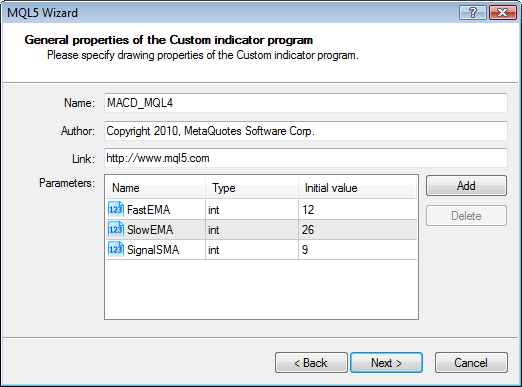Figure 1. Input parameters of the MACD indicator

Then create indicator buffers (fig. 2) according to what is written about them in the MQL4 program:

```//---- indicator buffers
double     MacdBuffer[];
double     SignalBuffer[];```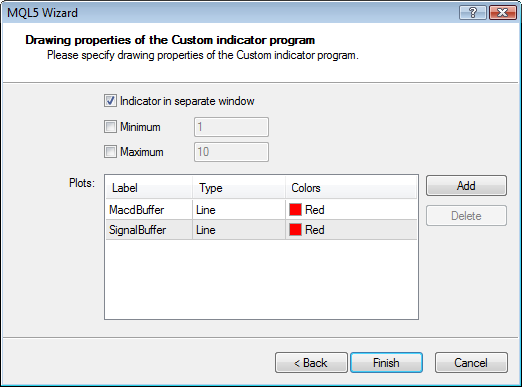Figure 2. The indicator buffers of MACD

Now we have formed a template for our new indicator.

You need to make several modifications to it. Add a line above the input parameters:

```#include <mql4_2_mql5.mqh>
//--- input parameters
```

To the function:

`int OnInit()`

`    InitMql4();`
and add a line that is responsible for starting the environment for the MQL4 program to the body of the program:
```int bars=MQL4Run(rates_total,prev_calculated);
// bars - number of bars available to MQL4 programs```

As you can see, this function will return number of bars available for the MQL4 environment; a new variable appears here as well:

`int CountedMQL4;`

This variable is an analog of the MQL5 variable

` prev_calculated,`

The CountedMQL4 variable is declared in the included file; it passes the amount of calculated data.

Then insert our prepared MQL4 section into the formed MQL5 template after the last symbol.

Now we need to start the indicator.

To do it, add the following line to the body of the program:

```Start(bars,
CountedMQL4,
MacdBuffer,
SignalBuffer,
FastEMA,
SlowEMA,
SignalSMA);```

As you see, this line will pass data, that is necessary for our MQL4 program, as well as the links to resulting buffers, which names we will take from the template created in MQL5.

The following result should be obtained:

```//+------------------------------------------------------------------+
//|                                                     MACD_MQ4.mq5 |
//|                        Copyright 2010, MetaQuotes Software Corp. |
//|                                              http://www.mql5.com |
//+------------------------------------------------------------------+
#property version   "1.00"
#property indicator_separate_window
#property indicator_buffers 2
#property indicator_plots   2
//--- plot MacdBuffer
#property indicator_label1  "MacdBuffer"
#property indicator_type1   DRAW_LINE
#property indicator_color1  Red
#property indicator_style1  STYLE_SOLID
#property indicator_width1  1
//--- plot SignalBuffer
#property indicator_label2  "SignalBuffer"
#property indicator_type2   DRAW_LINE
#property indicator_color2  Red
#property indicator_style2  STYLE_SOLID
#property indicator_width2  1
//--- input parameters
#include <mql4_2_mql5.mqh>
input int      FastEMA=12;
input int      SlowEMA=26;
input int      SignalSMA=9;
//--- indicator buffers
double         MacdBuffer[];
double         SignalBuffer[];
//+------------------------------------------------------------------+
//| Custom indicator initialization function                         |
//+------------------------------------------------------------------+
int OnInit()
{
//--- indicator buffers mapping
SetIndexBuffer(0,MacdBuffer,INDICATOR_DATA);
SetIndexBuffer(1,SignalBuffer,INDICATOR_DATA);
//---
InitMql4();
//---
return(0);
}
//+------------------------------------------------------------------+
//| Custom indicator iteration function                              |
//+------------------------------------------------------------------+
int OnCalculate(const int rates_total,
const int prev_calculated,
const datetime& time[],
const double& open[],
const double& high[],
const double& low[],
const double& close[],
const long& tick_volume[],
const long& volume[],
{
//---
int bars=MQL4Run(rates_total,prev_calculated);
// bars - number of bars available to MQL4 programs

Start(bars,
CountedMQL4,
MacdBuffer,
SignalBuffer,
FastEMA,
SlowEMA,
SignalSMA);//--- return value of prev_calculated for next call
return(rates_total);
}
//+------------------------------------------------------------------+
//| Moving Averages Convergence/Divergence                           |
//+------------------------------------------------------------------+
int Start(int rates_total,
int prev_calculated,
double &MacdBuffer[],
double &SignalBuffer[],
int FastEMA,
int SlowEMA,
int SignalSMA)
{
int limit;
int counted_bars=IndicatorCounted();
//---- last counted bar will be recounted
if(counted_bars>0) counted_bars--;
limit=Bars-counted_bars;
//---- macd counted in the 1-st buffer
for(int i=0; i<limit; i++)
MacdBuffer[i]=iMA(NULL,0,FastEMA,0,MODE_EMA,PRICE_CLOSE,i)
-iMA(NULL,0,SlowEMA,0,MODE_EMA,PRICE_CLOSE,i);
//---- signal line counted in the 2-nd buffer
for(i=0; i<limit; i++)
SignalBuffer[i]=iMAOnArray(MacdBuffer,Bars,SignalSMA,0,MODE_SMA,i);
//---- done
return(0);
}```

This is just the first stage of the transfer; now we start debugging the indicator.

3. Peculiarities of Working with Indicator Buffers in MQL5

Since many predefined variables of MQL4 correspond to the names of predefined variables of MQL5, you should make the following changes to the transferred MQL4 section:

MQL4
MQL5
IndicatorCounted()
prev_calculated
Bars rates_total
iMA( iMAMql4(
iMAOnArray( iMAOnArrayMql4(

```//+--------------------+------------------+
//|              MQL4  | MQL5             |
//+--------------------+------------------+
//|IndicatorCounted()  | prev_calculated  |
//|              Bars  | rates_total      |
//|              iMA(  | iMAMql4(         |
//|       iMAOnArray(  | iMAOnArrayMql4(  |
//+--------------------+------------------+ ```

Regarding the peculiarities of organization of data storage, the MQL5 reference says the following about SetIndexBuffer():

Note

After binding, the dynamic array buffer[] will be indexed as in common arrays, even if the indexing of timeseries is pre-installed for the bound array . If you want to change the order of access to elements of the indicator array, use the ArraySetAsSeries() function after binding the array using the SetIndexBuffer() function.

Thus, the initial policy of accessing indicator buffers now corresponds to working with ordinary arrays, so you should always add the binding:

```   ArraySetAsSeries(MacdBuffer,true);
ArraySetAsSeries(SignalBuffer,true);```

The resultant code is the following:

```//+------------------------------------------------------------------+
//|                                                    MACD_MQL4.mq5 |
//|                        Copyright 2010, MetaQuotes Software Corp. |
//|                                              http://www.mql5.com |
//+------------------------------------------------------------------+
#property version   "1.00"
#property indicator_separate_window
#property indicator_buffers 2
#property indicator_plots   2
//--- plot MacdBuffer
#property indicator_label1  "MacdBuffer"
#property indicator_type1   DRAW_LINE
#property indicator_color1  Red
#property indicator_style1  STYLE_SOLID
#property indicator_width1  1
//--- plot SignalBuffer
#property indicator_label2  "SignalBuffer"
#property indicator_type2   DRAW_LINE
#property indicator_color2  Red
#property indicator_style2  STYLE_SOLID
#property indicator_width2  1

#include <mql4_2_mql5.mqh>
//--- input parameters
input int      FastEMA=12;
input int      SlowEMA=26;
input int      SignalSMA=9;
//--- indicator buffers
double         MacdBuffer[];
double         SignalBuffer[];
//+------------------------------------------------------------------+
//| Custom indicator initialization function                         |
//+------------------------------------------------------------------+
int OnInit()
{
//--- indicator buffers mapping
SetIndexBuffer(0,MacdBuffer,INDICATOR_DATA);
SetIndexBuffer(1,SignalBuffer,INDICATOR_DATA);
//---
InitMql4();
//---
ArraySetAsSeries(MacdBuffer,true);
ArraySetAsSeries(SignalBuffer,true);
//---
return(0);
}
//+------------------------------------------------------------------+
//| Custom indicator iteration function                              |
//+------------------------------------------------------------------+
int OnCalculate(const int rates_total,
const int prev_calculated,
const datetime &time[],
const double &open[],
const double &high[],
const double &low[],
const double &close[],
const long &tick_volume[],
const long &volume[],
{
//---
int bars=MQL4Run(rates_total,prev_calculated);
// bars - number of bars available for MQL4 programs
Start(bars,
CountedMQL4,
MacdBuffer,
SignalBuffer,
FastEMA,
SlowEMA,
SignalSMA);
//--- return value of prev_calculated for next call
return(rates_total);
}
//+------------------------------------------------------------------+
//| Moving Averages Convergence/Divergence                           |
//+------------------------------------------------------------------+
//+--------------------+------------------+
//|              MQL4  | MQL5             |
//+--------------------+------------------+
//|IndicatorCounted()  | prev_calculated  |
//|              Bars  | rates_total      |
//|              iMA(  | iMAMql4(         |
//|       iMAOnArray(  | iMAOnArrayMql4(  |
//+--------------------+------------------+
int Start(int rates_total,
int prev_calculated,
double &MacdBuffer[],
double &SignalBuffer[],
int FastEMA,
int SlowEMA,
int SignalSMA)
{
int limit;
int counted_bars=prev_calculated;
//---- last counted bar will be recounted
if(counted_bars>0) counted_bars--;
limit=rates_total-counted_bars;
//---- macd counted in the 1-st buffer
for(int i=0; i<limit; i++)
MacdBuffer[i]=iMAMql4(NULL,0,FastEMA,0,MODE_EMA,PRICE_CLOSE,i)
-iMAMql4(NULL,0,SlowEMA,0,MODE_EMA,PRICE_CLOSE,i);
//---- signal line counted in the 2-nd buffer
for(int i=0; i<limit; i++)
SignalBuffer[i]=iMAOnArrayMql4(MacdBuffer,rates_total,SignalSMA,0,MODE_SMA,i);
//---- done
return(0);
}
//+------------------------------------------------------------------+```

The result of execution is shown in the fig. 3: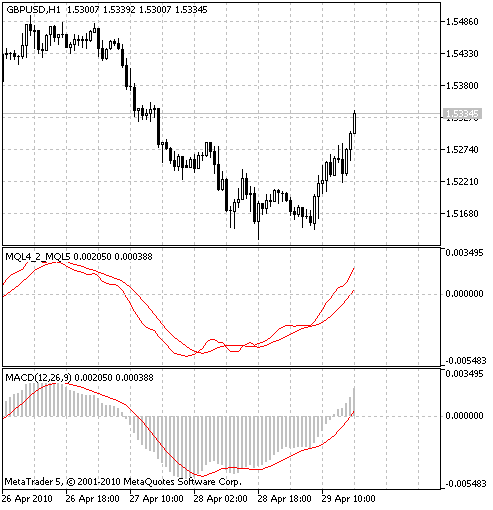Figure 3. Comparison of the MACD indicator rewritten from MQL4 and the standard one in MQL5.

4. Example of Transferring the Stochastic Indicator

Let's create a new template for our indicator in MetaEditor 5 (fig. 4-5):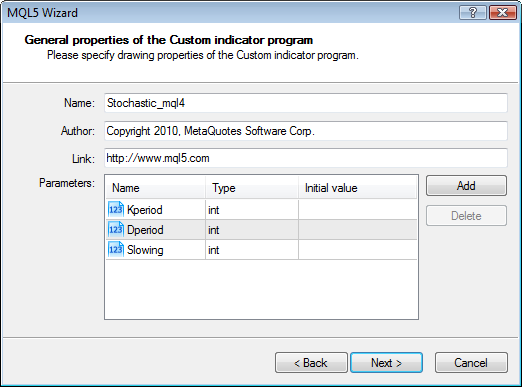Figure 4. Input parameters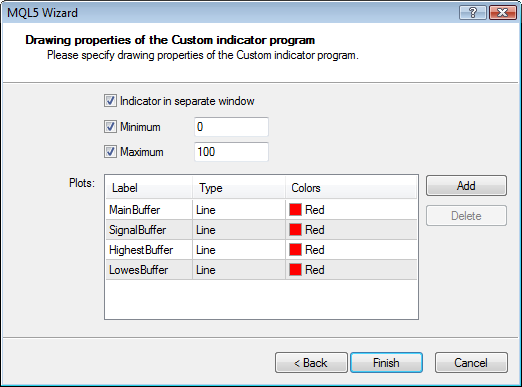Figure 5. Buffers

During debugging we figured out that several calculations from the MQL4 "OnInit" function should be moved inside the "Start" function by simple copying:

```int draw_begin1=KPeriod+Slowing;
int draw_begin2=draw_begin1+DPeriod;```
Also we need to change the number of buffers for drawing since in our MQL4 program 2 buffers are used for internal calculations and another 2 are used for drawing.
`#property indicator_plots   2`

And change the status of buffers that will be used by our MQL4 program for internal calculations.

```   SetIndexBuffer(2,HighesBuffer,INDICATOR_CALCULATIONS);
SetIndexBuffer(3,LowesBuffer,INDICATOR_CALCULATIONS);```
Make the necessary changes:
```//+------------------------------------------------------------------+
//|                                              Stochastic_MQL4.mq5 |
//|                        Copyright 2010, MetaQuotes Software Corp. |
//|                                              http://www.mql5.com |
//+------------------------------------------------------------------+
#property version   "1.00"
#property indicator_separate_window
#property indicator_minimum 0
#property indicator_maximum 100
#property indicator_buffers 4
#property indicator_plots   2
//--- plot MainBuffer
#property indicator_label1  "MainBuffer"
#property indicator_type1   DRAW_LINE
#property indicator_color1  Red
#property indicator_style1  STYLE_SOLID
#property indicator_width1  1
//--- plot SignalBuffer
#property indicator_label2  "SignalBuffer"
#property indicator_type2   DRAW_LINE
#property indicator_color2  Red
#property indicator_style2  STYLE_SOLID
#property indicator_width2  1

#include <mql4_2_mql5.mqh>
//--- input parameters
input int      Kperiod=14;
input int      Dperiod=5;
input int      Slowing=5;
//--- indicator buffers
double         MainBuffer[];
double         SignalBuffer[];
double         HighesBuffer[];
double         LowesBuffer[];
//+------------------------------------------------------------------+
//| Custom indicator initialization function                         |
//+------------------------------------------------------------------+
int OnInit()
{
//--- indicator buffers mapping
SetIndexBuffer(0,MainBuffer,INDICATOR_DATA);
SetIndexBuffer(1,SignalBuffer,INDICATOR_DATA);
SetIndexBuffer(2,HighesBuffer,INDICATOR_CALCULATIONS);
SetIndexBuffer(3,LowesBuffer,INDICATOR_CALCULATIONS);
//---
InitMql4();
//---
ArraySetAsSeries(MainBuffer,true);
ArraySetAsSeries(SignalBuffer,true);
ArraySetAsSeries(HighesBuffer,true);
ArraySetAsSeries(LowesBuffer,true);
//---
return(0);
}
//+------------------------------------------------------------------+
//| Custom indicator iteration function                              |
//+------------------------------------------------------------------+
int OnCalculate(const int rates_total,
const int prev_calculated,
const datetime &time[],
const double &open[],
const double &high[],
const double &low[],
const double &close[],
const long &tick_volume[],
const long &volume[],
{
//---
int bars=MQL4Run(rates_total,prev_calculated);
// bars - количество баров, доступных mql4-программам
start(bars,
CountedMQL4,
MainBuffer,
SignalBuffer,
HighesBuffer,
LowesBuffer,
Kperiod,
Dperiod,
Slowing);
//--- return value of prev_calculated for next call
return(rates_total);
}
//+--------------------+------------------+
//|              MQL4  | MQL5             |
//+--------------------+------------------+
//|IndicatorCounted()  | prev_calculated  |
//|              Bars  | rates_total      |
//|              iMA(  | iMAMql4(         |
//|       iMAOnArray(  | iMAOnArrayMql4(  |
//+--------------------+------------------+
int start(int rates_total,
int prev_calculated,
double &MainBuffer[],
double &SignalBuffer[],
double &HighesBuffer[],
double &LowesBuffer[],
int KPeriod,
int DPeriod,
int Slowing)
{
int draw_begin1=KPeriod+Slowing;
int draw_begin2=draw_begin1+DPeriod;
int    i,k;
int    counted_bars=prev_calculated;
double price;
//----
if(rates_total<=draw_begin2) return(0);
//---- initial zero
if(counted_bars<1)
{
for(i=1;i<=draw_begin1;i++) MainBuffer[rates_total-i]=0;
for(i=1;i<=draw_begin2;i++) SignalBuffer[rates_total-i]=0;
}
//---- minimums counting
i=rates_total-KPeriod;
if(counted_bars>KPeriod) i=rates_total-counted_bars-1;
while(i>=0)
{
double min=1000000;
k=i+KPeriod-1;
while(k>=i)
{
price=Low[k];
if(min>price) min=price;
k--;
}
LowesBuffer[i]=min;
i--;
}
//---- maximums counting
i=rates_total-KPeriod;
if(counted_bars>KPeriod) i=rates_total-counted_bars-1;
while(i>=0)
{
double max=-1000000;
k=i+KPeriod-1;
while(k>=i)
{
price=High[k];
if(max<price) max=price;
k--;
}
HighesBuffer[i]=max;
i--;
}
//---- %K line
i=rates_total-draw_begin1;
if(counted_bars>draw_begin1) i=rates_total-counted_bars-1;
while(i>=0)
{
double sumlow=0.0;
double sumhigh=0.0;
for(k=(i+Slowing-1);k>=i;k--)
{
sumlow+=Close[k]-LowesBuffer[k];
sumhigh+=HighesBuffer[k]-LowesBuffer[k];
}
if(sumhigh==0.0) MainBuffer[i]=100.0;
else MainBuffer[i]=sumlow/sumhigh*100;
i--;
}
//---- last counted bar will be recounted
if(counted_bars>0) counted_bars--;
int limit=rates_total-counted_bars;
//---- signal line is simple moving average
for(i=0; i<limit; i++)
SignalBuffer[i]=iMAOnArrayMql4(MainBuffer,rates_total,DPeriod,0,MODE_SMA,i);
//----
return(0);
}
//+------------------------------------------------------------------+```

As a result we obtained a full-fledged stochastic in MQL5 with the MQL4 price constructions.

The result of its working is shown in the fig. 6: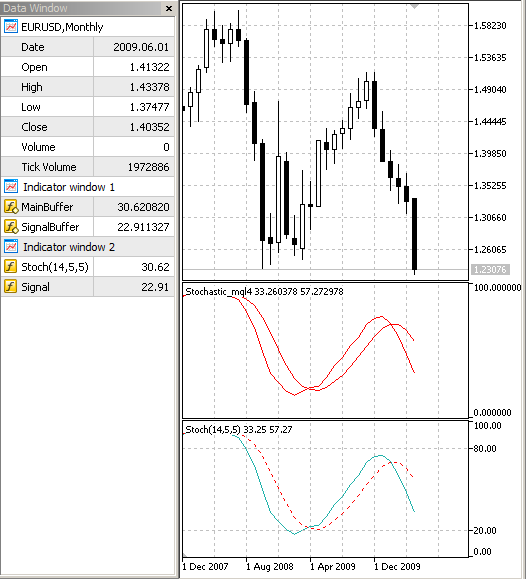Figure 6. Comparison of the indicator Stochastic rewritten from MQL4 and the standard Stochastic in MQL5.

5. Example of Transferring the RSI Indicator

```//---- input parameters
extern int RSIPeriod=14;
//---- buffers
double RSIBuffer[];
double PosBuffer[];
double NegBuffer[];```

And create a template for it in MetaEditor 5 (fig. 7-8).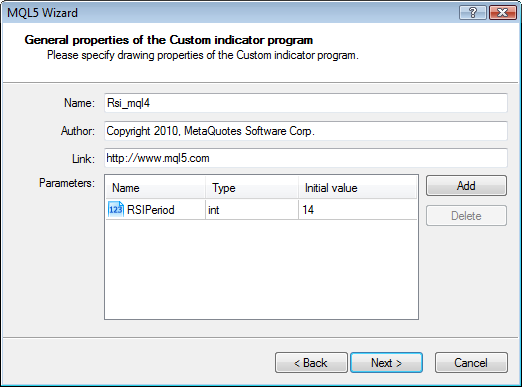Figure 7. Input parameter of the RSI indicator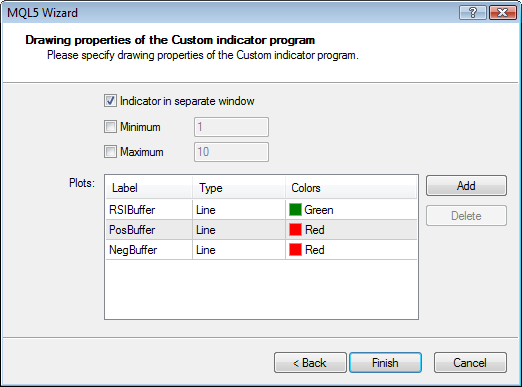Figure 8. Buffers of the RSI indicator

Total number of buffers is 3:

`#property indicator_buffers 3`

Number of buffers for plotting is equal to one:

`#property indicator_plots   1`

Set the status of buffers for calculations:

```   SetIndexBuffer(1,PosBuffer,INDICATOR_CALCULATIONS);
SetIndexBuffer(2,NegBuffer,INDICATOR_CALCULATIONS)```

Arrange the parts and make necessary changes:

```//+------------------------------------------------------------------+
//|                                                     RSI_MQL4.mq5 |
//|                        Copyright 2010, MetaQuotes Software Corp. |
//|                                              http://www.mql5.com |
//+------------------------------------------------------------------+
#property version   "1.00"
#property indicator_separate_window
#property indicator_buffers 3
#property indicator_plots   1
//--- plot RSIBuffer
#property indicator_label1  "RSIBuffer"
#property indicator_type1   DRAW_LINE
#property indicator_color1  Green
#property indicator_style1  STYLE_SOLID
#property indicator_width1  1
//--- plot PosBuffer
#property indicator_label2  "PosBuffer"
#property indicator_type2   DRAW_LINE
#property indicator_color2  Red
#property indicator_style2  STYLE_SOLID
#property indicator_width2  1
//--- plot NegBuffer
#property indicator_label3  "NegBuffer"
#property indicator_type3   DRAW_LINE
#property indicator_color3  Red
#property indicator_style3  STYLE_SOLID
#property indicator_width3  1

#include <mql4_2_mql5.mqh>
//--- input parameters
input int      RSIPeriod=14;
//--- indicator buffers
double         RSIBuffer[];
double         PosBuffer[];
double         NegBuffer[];
//+------------------------------------------------------------------+
//| Custom indicator initialization function                         |
//+------------------------------------------------------------------+
int OnInit()
{
//--- indicator buffers mapping
SetIndexBuffer(0,RSIBuffer,INDICATOR_DATA);
SetIndexBuffer(1,PosBuffer,INDICATOR_CALCULATIONS);
SetIndexBuffer(2,NegBuffer,INDICATOR_CALCULATIONS);
//---
InitMql4(3);

ArraySetAsSeries(RSIBuffer,true);
ArraySetAsSeries(PosBuffer,true);
ArraySetAsSeries(NegBuffer,true);

return(0);
}
//+------------------------------------------------------------------+
//| Custom indicator iteration function                              |
//+------------------------------------------------------------------+
int OnCalculate(const int rates_total,
const int prev_calculated,
const datetime &time[],
const double &open[],
const double &high[],
const double &low[],
const double &close[],
const long &tick_volume[],
const long &volume[],
{
int bars=MQL4Run(rates_total,prev_calculated);
// bars - number of bars available for MQL4 programs
RSImql4(bars,
CountedMQL4,
RSIBuffer,
PosBuffer,
NegBuffer,
RSIPeriod);
return(rates_total);
}
//+--------------------+------------------+
//|              MQL4  | MQL5             |
//+--------------------+------------------+
//|IndicatorCounted()  | prev_calculated  |
//|              Bars  | rates_total      |
//|              iMA(  | iMAMql4(         |
//|       iMAOnArray(  | iMAOnArrayMql4(  |
//+--------------------+------------------+
int RSImql4(int rates_total,
int prev_calculated,
double &RSIBuffer[],
double &PosBuffer[],
double &NegBuffer[],
int RSIPeriod)
{
int    i,counted_bars=prev_calculated;
double rel,negative,positive;
//----fd
if(rates_total<=RSIPeriod) return(0);
//---- initial zero
if(counted_bars<1)
for(i=1;i<=RSIPeriod;i++) RSIBuffer[rates_total-i]=0.0;
//----
i=rates_total-RSIPeriod-1;
if(counted_bars>=RSIPeriod) i=rates_total-counted_bars-1;
while(i>=0)
{
double sumn=0.0,sump=0.0;
if(i==rates_total-RSIPeriod-1)
{
int k=rates_total-2;
//---- initial accumulation
while(k>=i)
{
rel=Close[k]-Close[k+1];
if(rel>0) sump+=rel;
else      sumn-=rel;
k--;
}
positive=sump/RSIPeriod;
negative=sumn/RSIPeriod;
}
else
{
//---- smoothed moving average
rel=Close[i]-Close[i+1];
if(rel>0) sump=rel;
else      sumn=-rel;
positive=(PosBuffer[i+1]*(RSIPeriod-1)+sump)/RSIPeriod;
negative=(NegBuffer[i+1]*(RSIPeriod-1)+sumn)/RSIPeriod;
}
PosBuffer[i]=positive;
NegBuffer[i]=negative;
if(negative==0.0) RSIBuffer[i]=0.0;
else RSIBuffer[i]=100.0-100.0/(1+positive/negative);
i--;
}
//----
return(0);
}
//+------------------------------------------------------------------+```

Here, unlike in the previous indicator, we changed the name: now instead of the usual int Start() function in MQL4

```int start()
{```

we use

`int RSImql4(`

in MQL5. Both the name of the function itself and the line where it is called in the MQL5 program are changed.

The result of working of the library is shown in the fig. 9.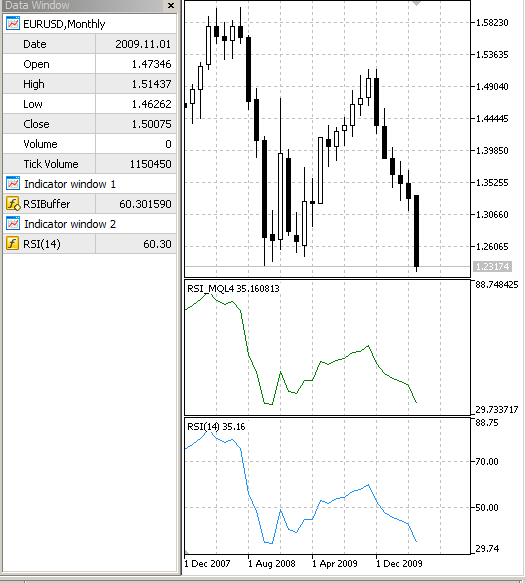Figure 9. Comparison of the RSIc indicator rewritten from MQL4 and the standard RSI indicator in MQL5.

6. Setup

To setup this module, you need to copy the mql4_2_mql5.mqh file to the MQL5\Include\ folder.

Test files should be placed to the MQL5\Indicators folder.

7. Improvement

If you want, you can extend the functionality of the module by connecting a library from the Migrating from MQL4 to MQL5 article. Add the InitMQL4.mqh file to the MQL5\Include folder and the following lines before the input parameters:

`#include <InitMQL4.mqh>`

You can get acquainted with the list of necessary changes in the Migrating from MQL4 to MQL5 article.

Conclusion

The algorithm of transferring simple price constructions from MQL4 to MQL5 using the special mql4_2_mql5.mqh library is represented in this article.

During debugging you may experience little troubles, but for the ones who are familiar with MQL4 it won't be a problem to cope with them.

Considering the peculiarities of accessing data in the MQL5 environment, recalculation of indicators may take some time. The reason is that we have to create and recalculate necessary data for programs from the MQL4 environment. For a full-fledged transfer of an indicator to the MQL5 environment it should be rewritten considering the peculiarities of storing and accessing data in MQL5.

Post Scriptum

I want to draw your attention to the phrase "Considering the peculiarities of accessing data in the MQL5 environment, recalculation of indicators may take some time. The reason is that we have to create and recalculate necessary data for programs from the MQL4 environment." Sometimes this waiting may last for several seconds (see fig. 10-11):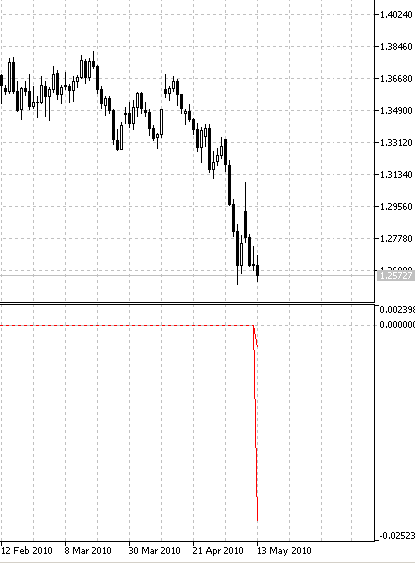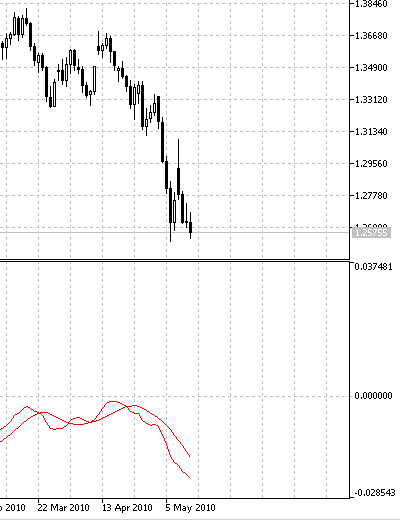Figure 10. Data is not calculated                                                                                                  Figure 11. Data is available

It is connected to a feature of the client terminal - only one copy of the calculation part is created in the cache of the terminal at creating handle of an indicator. If such indicator (with the same input parameters) is not created yet (), the call of the

`iMA(Symb,TimFram,iMAPeriod,ma_shift,ma_method,applied_price);`

function will create a moving average indicator; but only once.

Next time, at an attempt to create an already existing indicator, the terminal will just return its handle.

Thus, calculation of an indicator is performed only once, and not right after creation of its handle.

Translated from Russian by MetaQuotes Software Corp.
Original article: https://www.mql5.com/ru/articles/66

Attached files |
mql4_2_mql5.mqh (16.45 KB)
macd_mql4.mq5 (4.07 KB)
rsi_mql4.mq5 (4.77 KB)
Last comments | Go to discussion (3)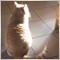| 14 Feb 2014 at 09:55| 18 Jan 2015 at 20:11
This library does not work. A pity as it seemed to be the more complete....dga | 16 Nov 2017 at 14:08

you can it esay  Zigzag   it have mql4  can Covert to mt5?

it Zigzag   Show next High TF for example  it Chart H4    Tf    but show Zigzag Daily to left h4

file Sorce Attachemt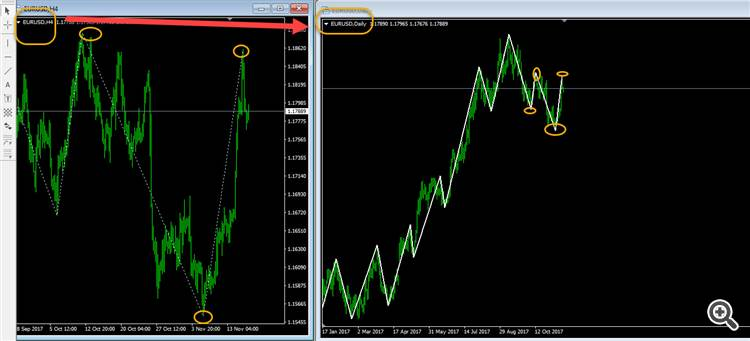Thanks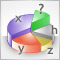The Optimal Method for Calculation of Total Position Volume by Specified Magic Number

The problem of calculation of the total position volume of the specified symbol and magic number is considered in this article. The proposed method requests only the minimum necessary part of the history of deals, finds the closest time when the total position was equal to zero, and performs the calculations with the recent deals. Working with global variables of the client terminal is also considered.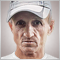Interview with Nikolay Kositsin: multicurrency EA are less risky (ATC 2010)

Nikolay Kositsin has told us about his developments. He believes multicurrency Expert Advisors are a promising direction; and he is an experienced developer of such robots. At the championships, Nikolay participates only with multicurrency EAs. His Expert Advisor was the only multicurrency EA among the prize winners of all the ATC contests.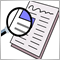Creating and Publishing of Trade Reports and SMS Notification

Traders don't always have ability and desire to seat at the trading terminal for hours. Especially, if trading system is more or less formalized and can automatically identify some of the market states. This article describes how to generate a report of trade results (using Expert Advisor, Indicator or Script) as HTML-file and upload it via FTP to WWW-server. We will also consider sending notification of trade events as SMS to mobile phone.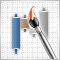Creating Multi-Colored Indicators in MQL5

In this article, we will consider how to create multi-colored indicators or convert the existing ones to multi-color. MQL5 allows to represent the information in the convenient form. Now it isn't necessary to look at a dozen of charts with indicators and perform analyses of the RSI or Stochastic levels, it's better just to paint the candles with different colors depending on the values of the indicators.